# Resources tagged with: Area - circles, sectors and segments

Filter by: Content type:
Age range:
Challenge level:

There are 27 NRICH Mathematical resources connected to Area - circles, sectors and segments, you may find related items under Measuring and calculating with units.

Broad Topics > Measuring and calculating with units > Area - circles, sectors and segments### Curvy Areas

##### Age 14 to 16Challenge Level

Have a go at creating these images based on circles. What do you notice about the areas of the different sections?### Partly Circles

##### Age 14 to 16Challenge Level

What is the same and what is different about these circle questions? What connections can you make?### Gutter

##### Age 14 to 16Challenge Level

Manufacturers need to minimise the amount of material used to make their product. What is the best cross-section for a gutter?### Spokes

##### Age 16 to 18Challenge Level

Draw three equal line segments in a unit circle to divide the circle into four parts of equal area.### Salinon

##### Age 14 to 16Challenge Level

This shape comprises four semi-circles. What is the relationship between the area of the shaded region and the area of the circle on AB as diameter?### An Unusual Shape

##### Age 11 to 14Challenge Level

Can you maximise the area available to a grazing goat?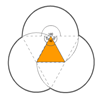### Triangles and Petals

##### Age 14 to 16Challenge Level

An equilateral triangle rotates around regular polygons and produces an outline like a flower. What are the perimeters of the different flowers?### Approximating Pi

##### Age 14 to 18Challenge Level

By inscribing a circle in a square and then a square in a circle find an approximation to pi. By using a hexagon, can you improve on the approximation?### Blue and White

##### Age 11 to 14Challenge Level

Identical squares of side one unit contain some circles shaded blue. In which of the four examples is the shaded area greatest?### Compare Areas

##### Age 14 to 16Challenge Level

Which has the greatest area, a circle or a square, inscribed in an isosceles right angle triangle?##### Age 14 to 16Challenge Level

Given a square ABCD of sides 10 cm, and using the corners as centres, construct four quadrants with radius 10 cm each inside the square. The four arcs intersect at P, Q, R and S. Find the. . . .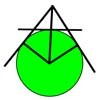### Mean Geometrically

##### Age 16 to 18Challenge Level

A and B are two points on a circle centre O. Tangents at A and B cut at C. CO cuts the circle at D. What is the relationship between areas of ADBO, ABO and ACBO?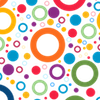### Circular Area

##### Age 7 to 11Challenge Level

How could you find out the area of a circle? Take a look at these ways.### Efficient Packing

##### Age 14 to 16Challenge Level

How efficiently can you pack together disks?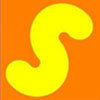### Two Shapes & Printer Ink

##### Age 14 to 16Challenge Level

If I print this page which shape will require the more yellow ink?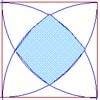### Bound to Be

##### Age 14 to 16Challenge Level

Four quadrants are drawn centred at the vertices of a square . Find the area of the central region bounded by the four arcs.### Squaring the Circle

##### Age 11 to 14Challenge Level

Bluey-green, white and transparent squares with a few odd bits of shapes around the perimeter. But, how many squares are there of each type in the complete circle? Study the picture and make. . . .### Crescents and Triangles

##### Age 14 to 16Challenge Level

Can you find a relationship between the area of the crescents and the area of the triangle?### Bull's Eye

##### Age 11 to 14Challenge Level

What fractions of the largest circle are the two shaded regions?### F'arc'tion

##### Age 14 to 16 ShortChallenge Level

At the corner of the cube circular arcs are drawn and the area enclosed shaded. What fraction of the surface area of the cube is shaded? Try working out the answer without recourse to pencil and. . . .### The Pillar of Chios

##### Age 14 to 16Challenge Level

Semicircles are drawn on the sides of a rectangle. Prove that the sum of the areas of the four crescents is equal to the area of the rectangle.### Floored

##### Age 14 to 16Challenge Level

A floor is covered by a tessellation of equilateral triangles, each having three equal arcs inside it. What proportion of the area of the tessellation is shaded?### Round and Round

##### Age 14 to 16Challenge Level

Prove that the shaded area of the semicircle is equal to the area of the inner circle.### Square Pegs

##### Age 11 to 14Challenge Level

Which is a better fit, a square peg in a round hole or a round peg in a square hole?### Two Circles

##### Age 14 to 16Challenge Level

Draw two circles, each of radius 1 unit, so that each circle goes through the centre of the other one. What is the area of the overlap?### Get Cross

##### Age 14 to 16Challenge Level

A white cross is placed symmetrically in a red disc with the central square of side length sqrt 2 and the arms of the cross of length 1 unit. What is the area of the disc still showing?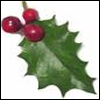### Giant Holly Leaf

##### Age 14 to 16Challenge Level

Find the perimeter and area of a holly leaf that will not lie flat (it has negative curvature with 'circles' having circumference greater than 2πr).